# 数据结构与算法—栈详解

### 什么是栈### 数组实现

``````private T data[];
private int top;
public seqStack() {
data=(T[]) new Object;
top=-1;
}
public seqStack(int maxsize)
{
data=(T[]) new Object[maxsize];
top=-1;
}
``````

push插入

• 如果top<数组长度-1。入栈,`top++;a[top]=value;`
• 如果top==数组长度-1；栈满。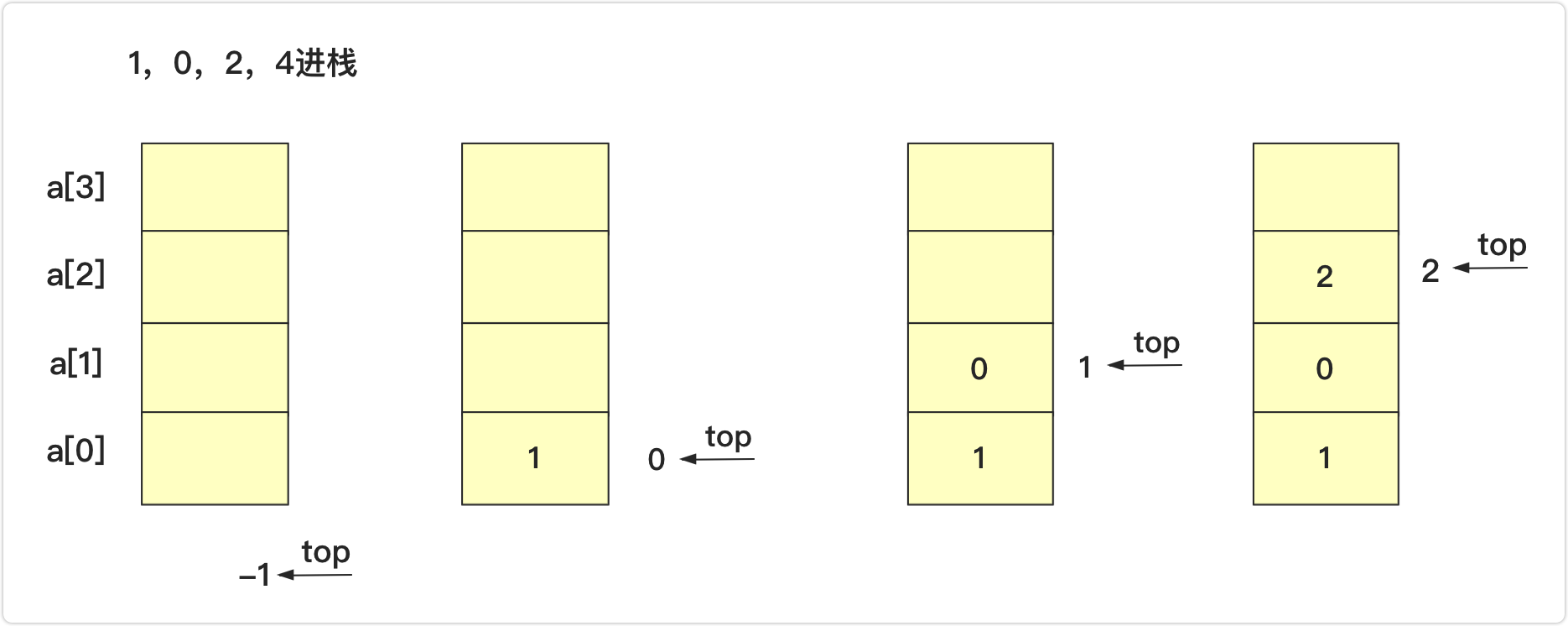pop弹出并返回首位

• 如果top>=0,栈不为空，可以弹出。`return data[top--];`
• 如下图，本来栈为1,2,3,4,5,6（栈顶）,执行pop操作,top变为3的位置并且返回4；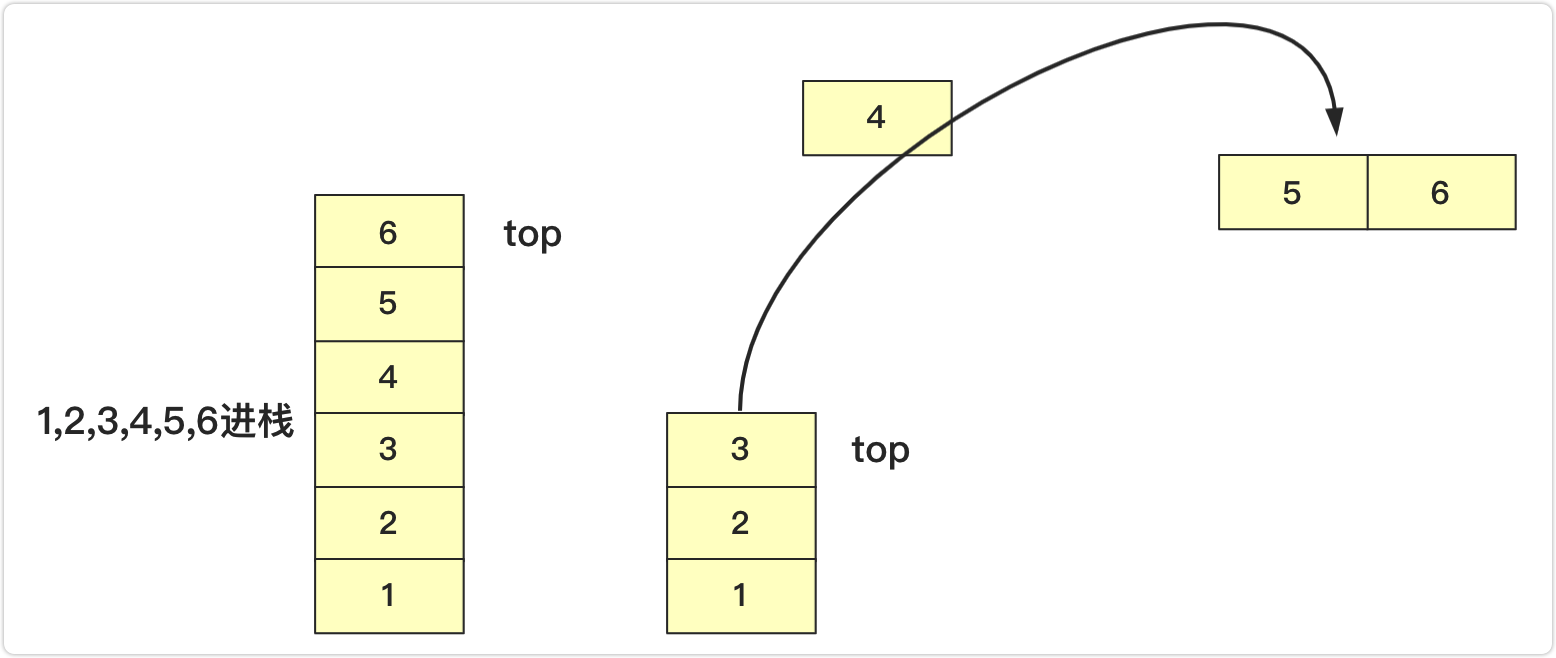``````package 队栈;

public class seqStack<T> {

private T data[];
private int top;
public seqStack() {
data=(T[]) new Object;
top=-1;
}
public seqStack(int maxsize)
{
data=(T[]) new Object[maxsize];
top=-1;
}
boolean isEmpty()
{
}
int length()
{
}

boolean push(T value) throws Exception//压入栈
{
if(top+1>data.length-1)
{
throw new Exception("栈已满");
}
else {
data[++top]=value;
return true;
}
}
T peek() throws Exception//返回栈顶元素不移除
{
if(!isEmpty())
{
return data[top];
}
else {
throw new Exception("栈为空");
}
}
T pop() throws Exception
{
if(isEmpty())
{
throw new Exception("栈为空");
}
else {
return data[top--];
}
}
public String toString()
{
if(top==-1)
{
return "";
}
else {
String va="";
for(int i=top;i>=0;i--)
{
va+=data[i]+"  ";
}
return va;
}
}
}

``````

### 链表实现

• 像数组那样在尾部插入删除。大家都知道链表效率低在查询，而查询到尾部效率很低，就算用了尾指针，可以解决尾部插入效率，但是依然无法解决删除效率(删除需要找到前驱节点)，还需要双向链表。前面虽然详细介绍过双向链表，但是这样未免太复杂
• 所以我们采用带头节点的单链表在头部插入删除，把头当成栈顶，插入直接在头节点后插入，删除也直接删除头节点后第一个节点即可，这样就可以完美的满足栈的需求。

``````static class node<T>
{
T data;
node next;
public node() {
}
public node(T value)
{
this.data=value;
}
}
``````

``````public class lisStack <T>{
int length;
public lisStack() {
length=0;
}
//其他方法
}
``````

push插入

• 空链表入栈`head.next=team;`
• 非空入栈`team.next=head.next;head.next=team;`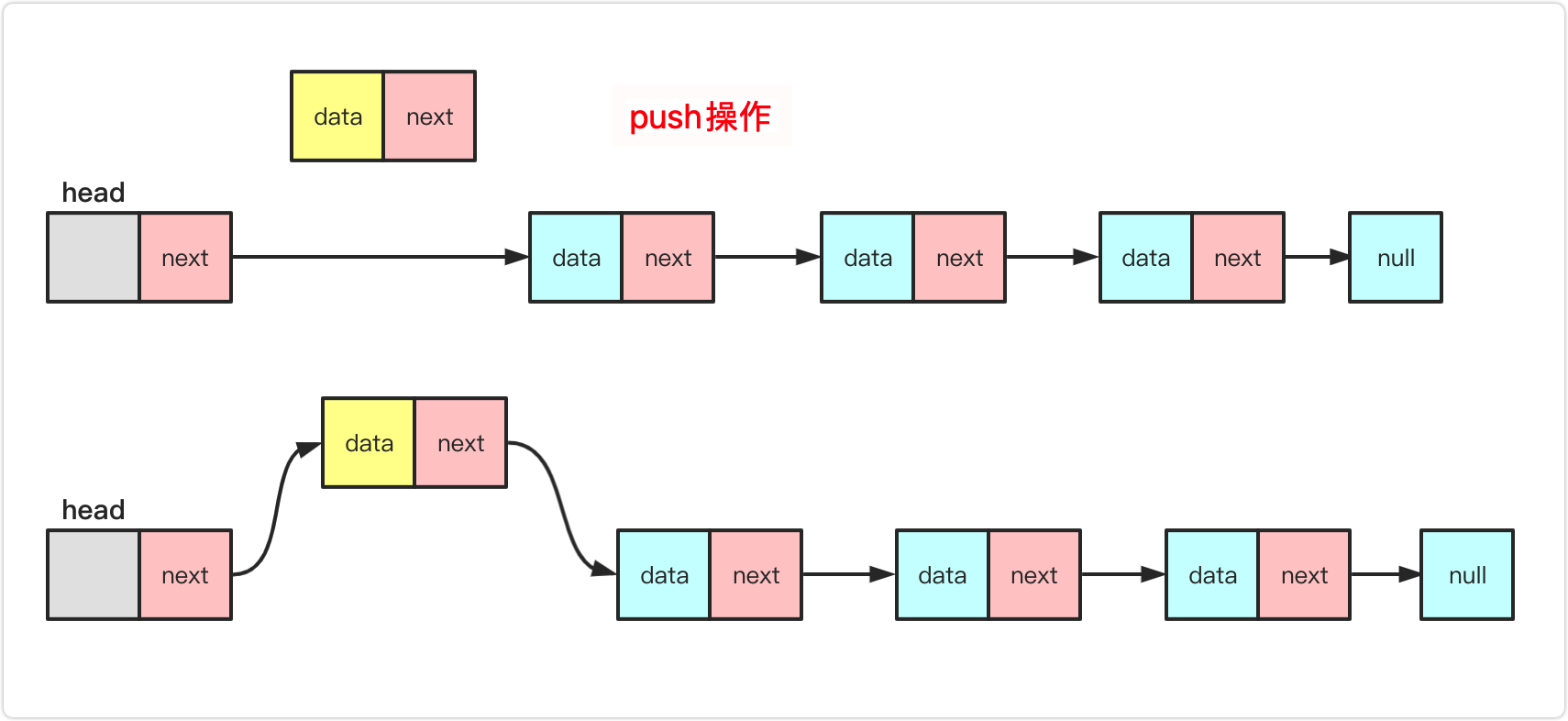pop弹出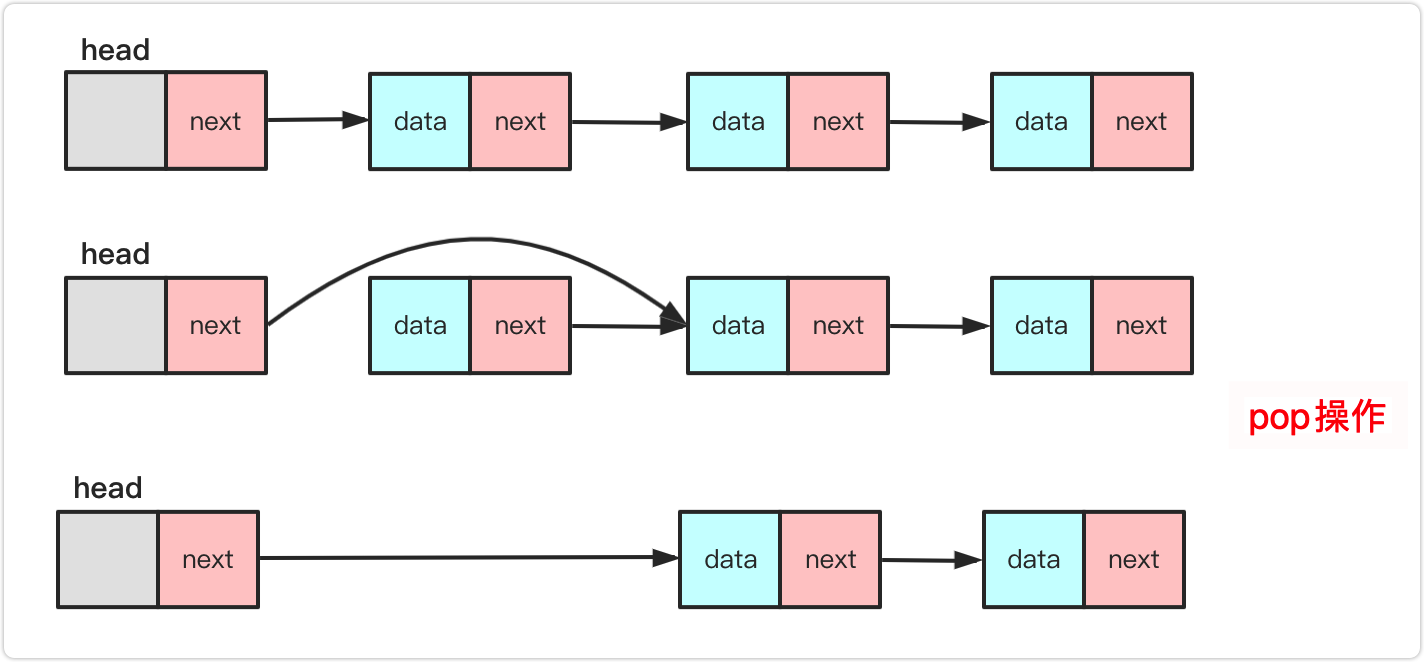``````package 队栈;

public class lisStack <T>{
static class node<T>
{
T data;
node next;
public node() {
}
public node(T value)
{
this.data=value;
}
}
int length;
public lisStack() {
length=0;
}
boolean isEmpty()
{
}
int length()
{
return length;
}
public void push(T value) {//近栈
node<T> team=new node<T>(value);
if(length==0)
{
}
else {
length++;
}
public T peek() throws Exception {
if(length==0) {throw new Exception("链表为空");}
else {//删除
}
}
public T pop() throws Exception {//出栈
if(length==0) {throw new Exception("链表为空");}
else {//删除
length--;
return value;
}
}
public String toString(){
if(length==0) {return "";}
else {
String va="";
while(team!=null)
{
va+=team.data+" ";
team=team.next;
}
return va;
}
}
}
``````

### 栈能这么玩

• `(({[(`下一个`)`消掉成`(({[`
• `(({[(`下一个`)`消掉成`(({[`
• `(({[`下一个`]`消掉成`(({`
• `(({`下一个`}`消掉成`((`
• `((`下一个`)`消掉成`(`
• `(`下一个`)`消掉成` ` 这样就满足题意

``````public boolean isValid(String s) {
Stack<Character>stack=new Stack<Character>();
for(int i=0;i<s.length();i++)
{
char te=s.charAt(i);
if(te==']')
{
if(!stack.isEmpty()&&stack.pop()=='[')
continue;
else {
return false;
}
}
else if(te=='}')
{
if(!stack.isEmpty()&&stack.pop()=='{')
continue;
else {
return false;
}
}
else if(te==')')
{
if(!stack.isEmpty()&&stack.pop()=='(')
continue;
else {
return false;
}
}
else
stack.push(te);
}
return stack.isEmpty();
}
``````

``````public boolean isValid(String s) {
char a[]=new char[s.length()];
int index=-1;
for(int i=0;i<s.length();i++)
{
char te=s.charAt(i);
if(te==']')
{
if(index>=0&&a[index]=='[')
index--;
else {
return false;
}
}
else if(te=='}')
{
if(index>=0&&a[index]=='{')
index--;
else {
return false;
}
}
else if(te==')')
{
if(index>=0&&a[index]=='(')
index--;
else {
return false;
}
}
else
a[++index]=te;
}
return index==-1;
}
``````

``````public  int longestValidParentheses(String s) {
char str[]=s.toCharArray();//字符数组
int max=0;
for(int i=0;i<str.length-1;i++)
{
int index=-1;
if(max>=str.length-i)
break;
for(int j=i;j<str.length;j++)
{
if(str[j]=='(')
index++;
else {
if(index<0)
{
i=j;
break;
}
else {
index--;
}
}
if(index==-1&&(j-i+1>max))
{
max=j-i+1;
}
}
}
return max;
}
``````

• `( ) ) ` `( ) ( ( ) ( ) )` 最大为后面部分(空格分开)
• `( ) ( ) ` `( ( ( )` 最大为前面部分
• `( ( ( ( ( ` `( ) ( ) ( ) ( )` 最大为后面部分

• 一种是当前已经超过左括号前面已经不可能连续了。例如`( ) ) ( )`第三个括号出现已经使得整个串串不可能连续，最大要么在其左面要么再其右面。 你可以理解其为一种清零初始机制。
• 另一种情况`)`就是目标栈中存在`(`可与其进行匹配。匹配之后要叠加到消除后平级的数量上，并且判断是否是最大值。(下面会解释)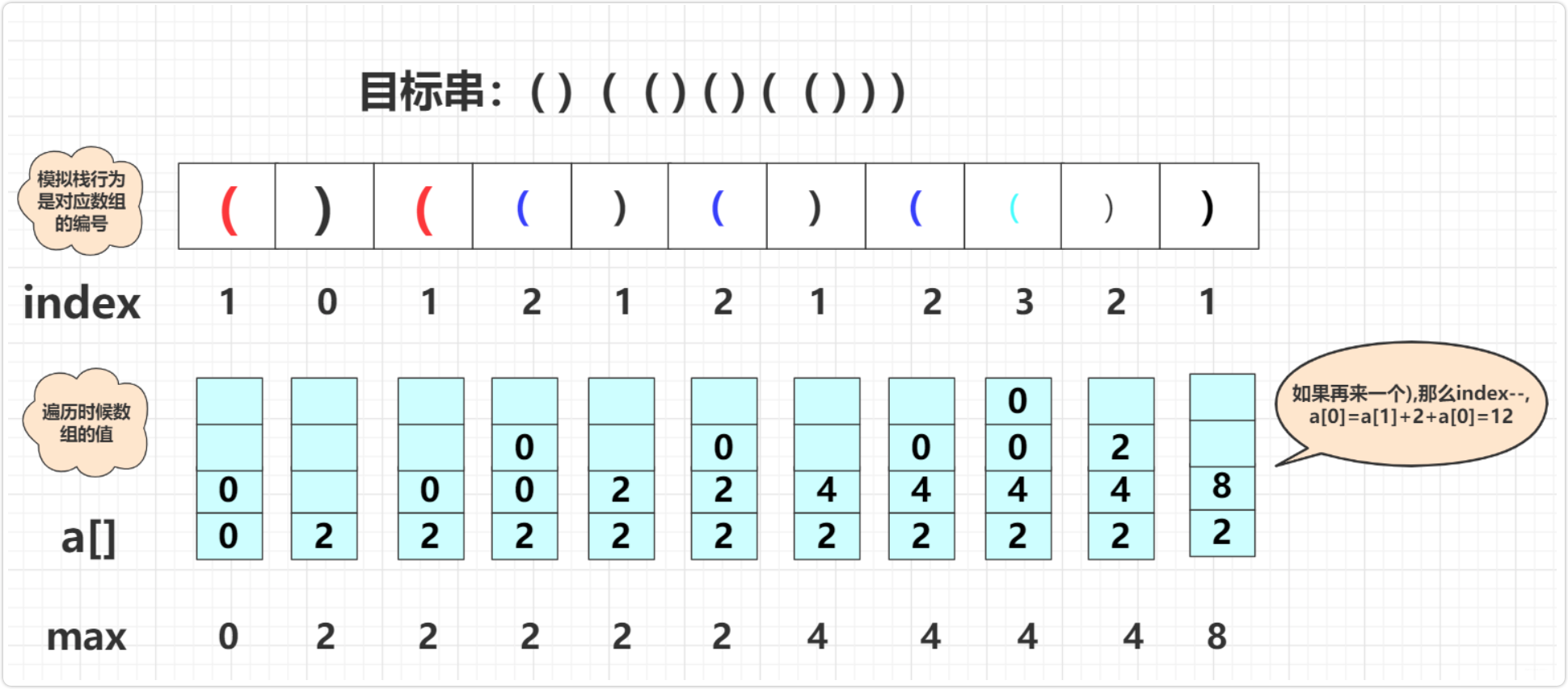`````` public static int longestValidParentheses(String s) {
int max=0;
int value[]=new int[s.length()+1];
int index=0;
for(int i=0;i<s.length();i++)
{
if(s.charAt(i)=='(')
{
index++;
value[index]=0;
}
else {//")"
if(index==0)
{
value=0;
}
else {
value[index-1]+=value[index--]+2;//叠加
if(value[index]>max)//更新
max=value[index];
}
}
}
return max;
}
``````

``````public int longestValidParentheses(String s) {
int maxans = 0;
Stack<Integer> stack = new Stack<>();
stack.push(-1);
for (int i = 0; i < s.length(); i++) {
if (s.charAt(i) == '(') {//(将当前的
stack.push(i);
} else {
stack.pop();
if (stack.empty()) {
stack.push(i);
} else {//i-stack.peek就是i是出现的总个数 peek是还没匹配的个数
maxans = Math.max(maxans, i - stack.peek());
}
}
}
return maxans;
}
``````

「其他文章」